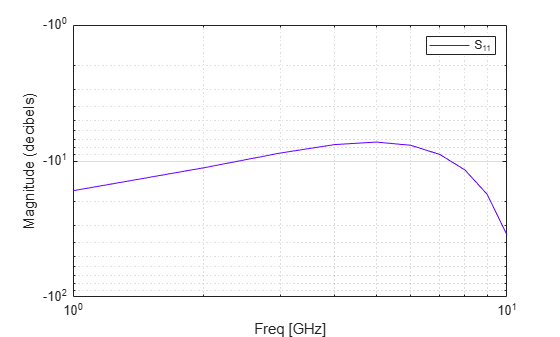# loglog

Plot specified circuit object parameters using log-log scale

## Syntax

``lineseries = loglog(h,parameter)``
``lineseries = loglog(h,parameter1,...,parametern)``
``lineseries = loglog(h,parameter1,...,parametern,format)``
``````lineseries=loglog(h,'parameter1',...,'parametern', format,xparameter ,xformat,'condition1',value1,..., 'conditionm',valuem,'freq',freq,'pin',pin)``````

## Description

````lineseries = loglog(h,parameter)` plots the specified `parameter` in the default format using a log-log scale. `h` is the handle of a circuit ( `rfckt` ) object.The `loglog` method returns a column vector of handles to `lineseries` objects, one handle per line. This output is the same as the output returned by the MATLAB® `loglog` method.```

example

````lineseries = loglog(h,parameter1,...,parametern)` plots the parameters `parameter1`, `...`, `parametern` from the object `h` on an X-Y plane using logarithmic scales for both the x- and y- axes.```
````lineseries = loglog(h,parameter1,...,parametern,format)` plots the parameters `parameter1`, `...`, `parametern` in the specified `format`. `format` is the format of the data to be plotted, e.g. `'Magnitude (decibels)'`. NoteFor all circuit objects except those that contain data from a data file, you must perform a frequency domain analysis with the `analyze` method before calling `loglog`. ```
``````lineseries=loglog(h,'parameter1',...,'parametern', format,xparameter ,xformat,'condition1',value1,..., 'conditionm',valuem,'freq',freq,'pin',pin)``` plots the specified parameters at the specified operating conditions for the object `h`. NoteIf `h` has multiple operating conditions, such as from a `.p2d` or `.s2d` file, the `loglog` method operates as follows: If you do not specify any operating conditions as arguments to the `loglog` method, then the method plots the parameter values based on the currently selected operating condition.If you specify one or more operating conditions, the `loglog` method plots the parameter values based on those operating conditions.When you use an operating condition for the `xparameter` input argument, the method plots the parameters for all operating condition values. ```

## Examples

collapse all

Create and analyze a two-wire network object.

``` tx1 = rfckt.twowire('Radius',7.5e-4); freq = linspace(1e9,10e9,10); analyze(tx1,freq)```
```ans = rfckt.twowire with properties: Radius: 7.5000e-04 Separation: 0.0016 MuR: 1 EpsilonR: 2.3000 LossTangent: 0 SigmaCond: Inf LineLength: 0.0100 StubMode: 'NotAStub' Termination: 'NotApplicable' nPort: 2 AnalyzedResult: [1x1 rfdata.data] Name: 'Two-Wire Transmission Line' ```

Plot S11 using the log-log scale.

` linesereis = loglog(tx1,'S11')````linesereis = Line (S_{11}) with properties: Color: [0 0.4470 0.7410] LineStyle: '-' LineWidth: 0.5000 Marker: 'none' MarkerSize: 6 MarkerFaceColor: 'none' XData: [1 2 3 4 5 6 7 8 9 10] YData: [1x10 double] ZData: [1x0 double] Show all properties ```

## Input Arguments

collapse all

RF circuit or data object, specified as object handle.

Data Types: `char` | `string`

Valid parameters of circuit objects, specified as a character vector or string.

Type `listparam(rfobject)` to get a list of valid parameters for a circuit object, `h`. Type `listformat(rfobject,parameter)` to see the legitimate formats for a specified `parameter`. The first listed format is the default for the specified parameter.

independent variable to use in plotting the specified parameters. Several `xparameter` values are available for all objects. When you import `rfckt.amplifier`, `rfckt.mixer`, or `rfdata.data` object specifications from a `.p2d` or `.s2d` file, you can also specify any operating conditions from the file that have numeric values, such as bias.

This table shows the commonly available parameters and the corresponding `xparameter` values. The default values listed in the table are used if the `xparameter` is not specified.

Parameter Name`xparameter` values
`Pout`, `Phase`, `LS11`, `LS12`, `LS21`, `LS22``Pin` (default), `Freq`
`S11`, `S12`, `S21`, `S22`, `NF`, `IIP3`, `OIP3`, `GroupDelay`, `VSWRIn`, `VSWROut`, `GammaIn`, `GammaOut`, `FMIN`, `GammaOPT`, `RN`, `TF1`, `TF2`, `Gt`, `Ga`, `Gp`, `Gmag`, `Gmsg`, `GammaMS`, `GammaML`, `K`, `Delta`, `Mu`, `MuPrime``Freq`
`AM/AM`, `AM/PM``AM`

Format for the specified `xparameter`. You do not need to specify `xformat` when `xparameter` is an operating condition.

This table shows the available `xformat` values that are available for the `xparameter` values, along with the default values that are used if `xformat` is not specified.

`xparameter` values`xformat` values
`Pin``dBm` (default), `mW`, `W`, `dBW`
`Freq`

`THz`, `GHz`, `MHz`, `KHz`, `Hz`

By default, `xformat` is chosen to provide the best scaling for the given `xparameter` values.

`AM``Magnitude (decibels)` (default), ```Magnitude (linear)```

### Name-Value Pair Arguments

Example: `semilogy(h,'Pout','Pin','Freq',2.1e9)`

Specify optional comma-separated pairs of `Name,Value` pair arguments. `Name` is the argument name and `Value` is the corresponding value. `Name` must appear inside single quotes (`''`). You can specify several name and value pair arguments in any order as `Name1, Value1, ..., NameN, ValueN`.

`'condition1',value1`, `...`, `conditionm,valuem` are the optional condition/value pairs at which to plot the specified parameters. These pairs are usually operating conditions from a `.p2d` or `.s2d` file. For some parameters, you can specify a set of frequency or input power values at which to plot the specified parameter.

For example:

• When plotting large-signal S-parameters as a function of input power, you can specify frequency points of interest using condition/value pairs.

• When plotting large-signal S-parameters as a function of frequency, you can specify input power levels of interest using condition/value pairs.

• When plotting parameters as a function of an operating condition, you can specify both frequency and input power values using condition/value pairs.

Frequency in Hz, specified as the comma-separated pair consisting of `'Freq'` and a positive scalar.

Input power level, in dBm, specified as the comma-separated pair consisting of `'Pin'` and a positive scalar.

## Output Arguments

collapse all

`lineseries` object, returned as a column vector of object handles.